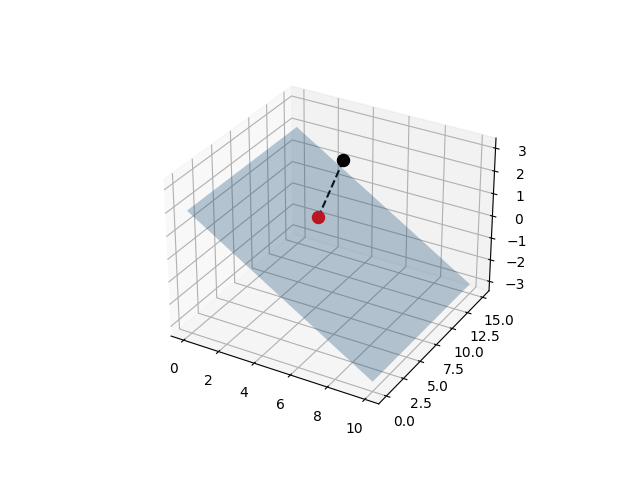# Point-Plane Projection¶

Project a point onto a plane.Out:

```(<Figure size 640x480 with 1 Axes>, <Axes3DSubplot:>)
```

```from skspatial.objects import Plane, Point, Vector
from skspatial.plotting import plot_3d

plane = Plane(point=[0, 0, 2], normal=[1, 0, 2])
point = Point([5, 9, 3])

point_projected = plane.project_point(point)
vector_projection = Vector.from_points(point, point_projected)

plot_3d(
plane.plotter(lims_x=(0, 10), lims_y=(0, 15), alpha=0.3),
point.plotter(s=75, c='k'),
point_projected.plotter(c='r', s=75, zorder=3),
vector_projection.plotter(point=point, c='k', linestyle='--'),
)
```

Total running time of the script: ( 0 minutes 0.168 seconds)

Gallery generated by Sphinx-Gallery